### Motion - Test Papers

CBSE Test Paper 01

Chapter 08 Motion

1. If a body is at rest, then its velocity-time graph is (1)

1.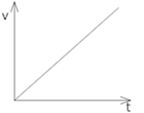2.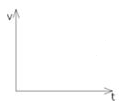3.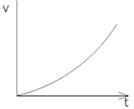4.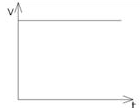2. Which of the is true for displacement? (1)
A) It cannot be zero
B) Its magnitude is greater than the distance travelled by the object.
C) It’s the shortest distance between initial & final position.
D) It is a vector quantity.

1. (A), (B) and (C) are correct
2. (A) and (B) are correct
3. All of these
4. (C) and (D) are correct
3. A moving body is covering a distance directly proportional to the square of time. The acceleration of the body is (1)

1. constant
2. zero
3. increasing
4. decreasing
4. Suppose a boy is enjoying a ride on a merry-go-round which is moving with a constant speed of 10ms-1. It implies that the boy is (1)

1. moving with no acceleration
2. at rest
3. in accelerated motion
4. moving with uniform velocity
5. What does the slope of velocity-time graph gives? (1)

1. acceleration
2. force
3. displacement
4. distance
6. What does the odometer of an automobile measure? (1)

7. State SI unit of acceleration. (1)

8. Give an example of non-uniform acceleration? (1)

9. Define the term "displacement". (1)

10. State SI unit of speed. (1)

11. Why rest and motion are called relative terms? (3)

12. A farmer moves along the boundary of a square field of side 10 m. in 40 s. What will be the magnitude of displacement of the farmer at the end of 2 minutes 20 seconds (3)

13. A stone is thrown in a vertically upward direction with a velocity of 5 ms-1. If the acceleration of the stone during its motion is 10 ms-2in the downward direction, what will be the height attained by the stone and how much time will it take to reach there? (3)

14. Two boys A and B, travel along the same path. The displacement - time graph for their journey is given in the following figure: (5)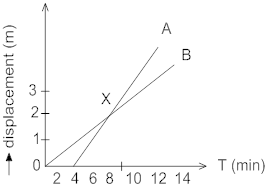1. How far down the road has B travelled when A starts the journey?
2. Without calculation, the speed, state who is travelling faster A or B?
3. What is the speed of A?
4. What is the speed of B?
5. Are the speed of A and B uniform?
6. What dose point X on the graph represent?
7. What is the speed of approach of A towards B?
8. What is the speed of separation of A from B?
15. Derive the second equation of motion S= ut +atgraphically? (5)

CBSE Test Paper 01
Chapter 08 Motion

1.Explanation: Velocity-time graph when the body is at rest: By saying that a body is a rest, it means that the velocity (v) of the body is zero at the time (t). So, the velocity-time graph of a body at rest is a straight line on the time axis.
1. (C) and (D) are correct
Explanation: When a body moves from one position to another,the shortest (straight line) distance between the initial position and final position of the body is known as its displacement. Its a vector quantity.
Displacement can be zero,less than the magnitude of distance but can never be more than the magnitude of distance covered.
1. constant
Explanation: Displacement is proportional to square of time
s is displacement and t is time then,
s ∝ t2
s = kt2
velocity(v) = 2kt
Acceleration(a) = 2k = constant.
So, the motion of body is constant acceleration.
1. in accelerated motion
Explanation: In a merry go round,the speed is constant but the velocity is not constant because its direction will be changing i.e, there is acceleration in the motion.So the boy is in accelerated motion.
1. acceleration
Explanation: We can find out the value of acceleration from the slope of velocity-time graph of a moving body.

Acceleration = $\frac{Change\phantom{\rule{thinmathspace}{0ex}}\phantom{\rule{thinmathspace}{0ex}}in\phantom{\rule{thinmathspace}{0ex}}\phantom{\rule{thinmathspace}{0ex}}velocity}{\phantom{\rule{thinmathspace}{0ex}}time}$ = Slope of the velocity-time graph provided.

1. The odometer measures the instantaneous speed of the automobile at some particular time.

2. S.I. unit of acceleration is metre / second2 (ms-2).

3. A car travelling along a straight road having much traffic increases or decreases its speed by unequal amounts in equal intervals of time.

4. The shortest distance between the initial and final positions of the object in a particular direction.
5. SI unit of speed is Metre / Second (ms-1).

6. Rest and motion are relative terms. A body may seem to be at rest with respect to one object, but may appear to be in motion with respect to another object. If you consider a passenger in a moving train, he is at rest with respect to his co-passengers, but is in motion with respect to an observer standing on the ground.

7. The perimeter of square field ABCD = 4 $×$ 10m = 40m
Time for moving around the 10 m square field once = 40 s.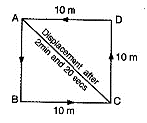Time for the journey of farmer = 2 min and 20 s = 140 s Number of times the farmer moves around the square field = $\begin{array}{l}\frac{140}{40}\end{array}$ = 3.5 times
For going once around the square field, the displacement = 0
For going thrice around the square field, the displacement = 0
For going 1/2 times the square field, the distance covered =40m $×$$\begin{array}{l}\frac{1}{2}\end{array}$m = 20m
It is obvious from the figure, that if the farmer starts from pt. A then he will cover 10 m along AB and then 10 m along BC.
Therefore displacement of a farmer from the pt. A to point C is
$\begin{array}{l}AC=\phantom{\rule{thickmathspace}{0ex}}\sqrt{B{C}^{2}\phantom{\rule{thickmathspace}{0ex}}+A{B}^{2}\phantom{\rule{thickmathspace}{0ex}}}=\phantom{\rule{thickmathspace}{0ex}}\sqrt{{10}^{2}\phantom{\rule{thickmathspace}{0ex}}+{10}^{2}\phantom{\rule{thickmathspace}{0ex}}}\phantom{\rule{thickmathspace}{0ex}}\phantom{\rule{thickmathspace}{0ex}}=14.14m\end{array}$

8. u = 5 ms-1, a = -10 ms-2
v = 0 (since at maximum height its velocity will be zero)
v = u + at = 5 + (-10) $×$ t
0 = 5 – 10t
10t = 5, or, t = 5/10 =0.5second.
s = ut + $\frac{1}{2}$at2= 5 $×$ 0.5 + $\frac{1}{2}$ $×$ (-10) $×$ 0.52
= 2.5 – 1.25 = 1.25m

1. When A starts his journey at 4 sec, B has already covered a distance of 857 m
2. A travels faster than B because A starts his journey late but crosses B and covers more distance then B in the same time as B
3. Speed of A =
Let at t =12 min, distance covered = 3500 m
$\frac{3500}{12}$ = 375 m/min
4. Speed of B =
${\mathrm{V}}_{B}=\frac{3000}{12}$ = 214 m/min
5. Speed of approach of A towards B = 375 m/min - 214 m/min = 161 m/min
6. Speed of separation of A from B = 161 m/min.
9.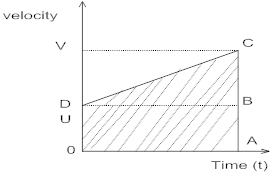Let at time T=0, body moves with initial velocity u and at time ‘t’ body has final velocity ‘v’ and in time ‘t’ it covers a distance 'S'.
AC = v, AB = u, OA = t, DB = OA = t, BC = AC - AB = v - u
Area under a v-t curve gives displacement so,
S= Area of triangle DBC + Area of rectangle OABD .......(i)
Area of $\mathrm{\Delta }DBC=\frac{1}{2}×Base×Height$
$\frac{1}{2}×DB×BC$
$\frac{1}{2}×t×\left(v-u\right)$ ......... (ii)
Area of rectangle OABD $=length×breadth$
$OA×BA$
$t×u$ ......... (iii)
From (i), (ii) and (iii)
S= ut+ $\frac{1}{2}×t×\left(v-u\right)$
S= ut + $\frac{1}{2}×t×at$
S= ut +